6461.0 - Australian Consumer Price Index: Concepts, Sources and Methods, 2005
ARCHIVED ISSUE Released at 11:30 AM (CANBERRA TIME) 17/08/2005
Page tools:Print All
Contents >> 9. Compiling the CPI

INTRODUCTION

9.1 The CPI has previously been described in terms of a basket of goods and services, which is 'purchased' each quarter. As prices change from one quarter to the next so too will the total cost (or price) of the basket. Of the various ways in which a CPI could be described, this description conforms closely with the procedures actually followed.

9.2 Using this description, the CPI can be thought of as being constructed in five major steps:

(i) subdividing the total expenditure into individual items for which price samples can be selected
(ii) collecting price data
(iii) estimating price movements for individual items
(iv) calculating the current period cost of the basket
(v) calculating index numbers and points contribution.

9.3 This Chapter provides a stylised account of the various steps above. It also indicates how analytical indexes are calculated and describes the ABS rounding practices.

SUBDIVIDING THE BASKET

Expenditure aggregates

9.4 Based mainly on the Household Expenditure Survey (HES) results, estimates are obtained for total annual expenditure of private households in each capital city for each of the 89 expenditure classes in the CPI. As these estimates relate to the expenditure of households in aggregate, they are referred to as ‘expenditure aggregates’.

9.5 Expenditure aggregates are derived for well-defined categories of household expenditure (e.g. bread), but are still too broad to be of direct use in selecting samples of products for pricing. For this purpose, expenditure aggregates need to be subdivided into as fine a level of commodity detail as possible. As the HES is generally not designed to provide such fine level estimates, it is necessary to supplement the HES data with information from other sources such as other official data collections and industry data. The processes involved are illustrated below by reference to a hypothetical example for the Bread expenditure class of the CPI.

9.6 Suppose that, based on information reported in the HES, the annual expenditure on bread by all private households in a particular city is estimated at \$8 million. Further, suppose that some industry data exists on the market shares of various types of bread. In combination, these two data sources can be used to derive expenditure aggregates at a much finer level of detail than that available from the HES alone. The hypothetical results are shown in table 9.1.

9.7 The next stage in the process involves determining the types of bread for which price samples should be constructed. This is not a simple exercise and relies heavily on the judgement of the prices statisticians. In reaching decisions about precisely which items to include in price samples, a balance needs to be struck between the cost of data collection (and processing) and the accuracy of the index. Factors taken into account include the relative significance of individual items, the extent to which different items are likely to exhibit similar price behaviour, and any practical problems associated with measuring prices to constant quality.

TABLE 9.1: DISAGGREGATION OF EXPENDITURE DATAType of bread Market Share HES data Derived expenditure aggregates% \$'000 \$'0001 White, sandwich, sliced 30 - 2,400 2 White, sandwich, unsliced 2 - 160 3 White high fibre 20 - 1,600 4 White high top 3 - 240 5 Wholemeal 10 - 800 6 Multigrain 15 - 1,200 7 Bread rolls 15 - 1,200 8 Specialty 5 - 400 Total Bread 100 8,000 8,000

9.8 In this example, a reasonable outcome would be to decide to construct pricing samples for varieties 1, 3, 5 and 6. Separate pricing samples would not be constructed for items 2 and 4 due to their relatively small market share. Pricing samples would also not be constructed for bread rolls and specialty breads (items 7 and 8) as they would prove difficult to price to constant quality due to the tendency for these items to be sold by number rather than weight.

Elementary aggregates must have a price sample

9.9 When no more information is available to further disaggregate the expenditure values, the resulting product definitions are called elementary aggregates. Each elementary aggregate has its own price sample. Ideally, all the products covered (and there should only be a few) would be homogeneous goods or services and would be substitutes for each other. In the Australian CPI there are approximately 1,000 elementary aggregates for each of the eight capital cities. This gives around 8,000 price samples at the national level. The expenditure aggregates for the items that are not explicitly priced are reallocated across the elementary aggregates of closely related goods or services under the assumption that the price movements for these products will be similar.

9.10 In the bread example, the reallocation would be carried out in two stages. First, the expenditure aggregate for unsliced white sandwich loaves would be added to sliced white sandwich loaves resulting in an elementary aggregate for white sandwich loaves (as it will be the characteristics of being white and sandwich loaves that make them likely to experience similar price movement pressures). White high fibre loaves would be treated similarly. In the second stage, the expenditure aggregates for bread rolls and speciality breads, which have no closely matching characteristics with any of the other types of bread, would be allocated, on a proportional basis, across the remaining elementary aggregates under the assumption that the average movement of prices for all other bread types will be the best estimate. The outcome of this process is presented in table 9.2.

9.11 In summary, the rationale for this allocation is as follows. Price behaviour of item 2 (white, sandwich, unsliced) is likely to be best represented by the price behaviour of item 1 (white, sandwich, sliced). Items 4 (white high top) and 3 (white high fibre) are treated similarly. The price behaviour for items 7 and 8 (bread rolls and specialty bread respectively) is likely to be best represented by the average price behaviour of all other breads.

TABLE 9.2: OUTCOME OF ELEMENTARY AGGREGATE RATIONALISATION
 Bread type Expenditure aggregates Elementary aggregateInitial Stage 1 Stage 2\$'000 \$'000 \$'0001 2,400 2,560 3,200 White sandwich 2 160 - - 3 1,600 1,840 2,300 White high fibre 4 240 - - 5 800 800 1,000 Wholemeal 6 1,200 1,200 1,500 Multigrain 7 1,200 1,200 -8 400 400 -Total 8,000 8,000 8,000Determining outlet types

9.12 Having settled on the product definitions for which price samples are to be constructed, the next step is to determine the outlet types (respondents) from which prices will be collected. In order to accurately reflect changes in prices paid by households for bread, prices need to be collected from the various types of outlets from which households purchase bread. Data are unlikely to be available on the expenditures at the individual elementary aggregate level by type of outlet. It is more likely that data will be available for expenditure on bread in total by type of outlet. Suppose industry data indicates that supermarkets accounted for about 80% of bread sales and bakery outlets the remainder. A simple way to construct a pricing sample for each elementary aggregate that is representative of household shopping patterns is to have a ratio of four supermarkets to every bakery.

COLLECTING PRICE DATA

Selecting respondents

9.13 When pricing samples have been determined, ABS field staff determine from which individual supermarkets and bakeries the prices will be collected. The individual outlets are chosen to be representative of the two types of outlets taking into account the demographics of the city and the numbers required for the sample. Prices will be collected from any particular respondent at the same time of each collection period (e.g. first Monday of each month).

Selecting items to price

9.14 When a pricing sample contains respondent standard specifications (refer to Chapter 8 for an explanation of this term) field staff will determine, in conjunction with the outlet management, which specific items are most representative of the required type of product. Using the bread example, at one outlet it might be decided that the 680 g sliced white sandwich loaf best represents white sandwich bread while at another outlet it might be a 700 g white sandwich loaf. Once selected, the same product will be priced at that respondent while ever it is the most representative example.

9.15 An important part of the price collection process is the continual monitoring of the items for quality change. In the bread example, quality change could occur with, say, a change in the size (weight) of the loaf of bread. In this case, the price movement directly attributable to the change in loaf size would be removed to derive a pure price movement for the loaf.

ESTIMATION OF PRICE MOVEMENTS FOR ELEMENTARY AGGREGATES

9.16 Price relatives are calculated for each price in the sample and the geometric mean of these is calculated. The ratio of the current period’s geometric mean of price relatives to the previous period’s geometric mean of price relatives provides the change in the average price for the elementary aggregate. Using the hypothetical bread sample, table 9.3 shows price relatives being used to estimate the price movement for bread. These estimates of price movements are used to revalue the expenditure aggregates to current period prices, by applying the period to period price movement to the previous period’ ;s expenditure aggregate for each elementary aggregate. The updated expenditure aggregate provides an estimate of the cost of acquiring the base period quantity of the elementary aggregate’s products in the current period. (footnote 1)

TABLE 9.3: ESTIMATING PRICE MOVEMENT FOR AN ELEMENTARY AGGREGATEPrice relatives inPeriod 1 Period 2 Price movementPer cent White sandwich loaf Supermarket A 1.025 1.045 2.0 Supermarket B 1.030 0.950 -7.8 Supermarket C 1.040 1.065 2.4 Supermarket D 0.980 1.100 12.2 Bakery 1.100 1.250 13.6 Geometric mean 1.034 1.075 4.0CALCULATING THE CURRENT COST OF THE BASKET

9.17 Once price movements are calculated for each item, a geometric mean is calculated, which is used to derive price-updated expenditure aggregates for each elementary aggregate. These are then summed to derive the current cost of the total (or any portion of the) basket of goods and services. Index numbers are calculated from the expenditure aggregates at every level of the index. Table 9.4 shows the calculation of the expenditure value for the total of bread (an expenditure class in the example).

TABLE 9.4: AGGREGATION OF EXPENDITURE AGGREGATES FOR EXPENDITURE CLASSElementary Expenditure Price Expenditure aggregate aggregate change aggregate Period 1 Period 1 to Period 2 Period 2 \$'000 Per cent \$'000 White sandwich 3,200 4.0 3,328 White high fibre 2,300 3.5 2,381 Wholemeal 1,000 0.0 1,000 Multigrain 1,500 1.7 1,526 Total 8,000 - 8,235

9.18 Once the expenditure aggregates for all the elementary aggregates have been calculated from the price movements, the expenditure aggregates for all higher level components of the index structure are calculated by summing the expenditure aggregates of their components. A cut-down version of a CPI structure, incorporating the bread example, is shown in table 9.5. Price movements in period 2 are used to update the expenditure aggregates.

CALCULATING INDEX NUMBERS AND POINTS CONTRIBUTIONS

9.19 Table 9.5 also shows the calculation of index numbers and points contribution. It has been assumed that index numbers already exist for the link period (June quarter 2000 for the 14th series CPI) and period 1. Assume the expenditure aggregate for Cereals has been calculated using the same method as that for Bread, so that the two can be added and a movement calculated for Bread and cereals. Similarly, assume the expenditure aggregates for period 2 have been calculated for Other Foods and Non-food, so that expenditure aggregates can be calculated for Food and All groups.

9.20 When a price index has not been linked, indexes for any component can be calculated simply by dividing the current period expenditure aggregate by its expenditure aggregate in the reference period (when the index is set to 100.0). However, the CPI has been linked several times since its reference base (1989–90) and the index numbers must be calculated from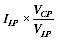(9.1)

where I LP is the index number in the link period (June quarter 2000 for the 14th series CPI), and VCP and VLP are the expenditure aggregates in the current period and link periods respectively. Thus the index number for Bread in period 2 is given by 108.0 x 8235 / 6500 = 136.8.

9.21 Points contributions are also calculated using the expenditure aggregates. In any period, the points contribution of a component to the All groups index number is calculated by multiplying the All groups index number for the period by the expenditure aggregate for the component in that period and dividing by the All groups expenditure aggregate for that period. This can be stated algebraically as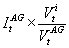(9.2)

where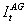is the index for All groups in period t,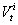is the expenditure aggregate for component i in period t and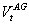is the expenditure aggregate for All groups in period t.

9.22 In the example in Table 9.5, the points contribution for Bread in period 2 is calculated as 141.3 * (8235 / 144268).

9.23 The change in index points contribution for a component between any two periods is found by simply subtracting the points contribution for the previous period from the points contribution for the current period. For example, the change in index points contribution for Bread between periods 1 and 2 is 8.07 - 7.84 = 0.23.

9.24 The CPI publication does not show the expenditure aggregates, but rather the index numbers derived from the expenditure aggregates. Expenditure aggregates vary considerably in size and showing them would make the publication difficult to read and interpret. The published index numbers and points contributions are a convenient presentation of the information.

TABLE 9.5: AGGREGATION OF EXPENDITURE AGGREGATES FOR ENTIRE INDEXLink period Period 1 Period 2Expenditure aggregatesAll groups 122500 138100 144268 Food 32500 40100 41368 Bread and cereals 12500 15000 15515 Bread 6500 8000 8235 Cereals 6000 7000 7280 Other foods 20000 25100 25853 Non-food 90000 98000 102900Movement in expenditure aggregates (period 1 to period 2)All groups1.045 Food1.032 Bread and cereals1.034 Bread1.029 Cereals1.040 Other foods1.030 Non-food1.050Index numbersAll groups 120.0 135.3 141.3 Food 115.0 141.9 146.4 Bread and cereals 110.0 132.0 136.5 Bread 108.0 132.9 136.8 Cereals 113.0 131.8 137.1 Other foods 117.0 146.8 151.2 Non-food 125.0 136.1 142.9Points contributionAll groups 120.0 135.3 141.3 Food 31.84 39.29 40.52 Bread and cereals 12.24 14.70 15.20 Bread 6.37 7.84 8.07 Cereals 5.88 6.86 7.13 Other foods 19.59 24.59 25.32 Non-food 88.16 96.01 100.78Note: it is assumed the reference base period precedes period 1.

SECONDARY INDEXES

9.25 A range of analytical indexes is published for the CPI. These include the ‘All groups excluding (each of the groups in turn)’ and ‘Goods and Services’ indexes. They are termed secondary indexes as they use the same weights (or expenditure aggregates) as the CPI - they are compiled from the summation of the appropriate value aggregates. For example, in Table 9.5 the starting point for compiling an index for All groups excluding Bread and cereals would be to add up the value aggregates for Other foods and Non food and then calculate index values as described in the previous section.

TERTIARY INDEXES

9.26 A further range of analytical indexes is compiled from the price samples collected for the CPI. Price indexes compiled under the outlays approach are produced annually for four population sub-groups: employees; age pensioners; self-funded retirees; and other government transfer recipients. These indexes, unlike the secondary indexes mentioned above, have their own weighting patterns and are compiled in a similar manner to the CPI. The purpose of the population sub-group indexes is to show any differences in the price changes faced by the four demographic groups, which would arise purely from their differing expenditure patterns.

CPI ROUNDING CONVENTIONS

9.27 To ensure consistency in the application of data produced from the CPI, it is necessary for the ABS to adopt a set of consistent rounding conventions or rules for calculating and presenting data. The conventions strike a balance between maximising the usefulness of the data for analytical purposes and retaining a sense of the underlying precision of the estimates. These conventions need to be taken into account when CPI data is used for analytical or other special purposes.

9.28 Index numbers are always published relative to a base of 100.0. Index numbers and percentage changes are always published to one decimal place, with the percentage changes being calculated from the rounded index numbers. Points contributions are published to two decimal places, with points contributions change being calculated from the rounded points contributions. Index numbers for periods longer than a single quarter (e.g. for financial years) are calculated as the simple arithmetic average of the relevant rounded quarterly index numbers.

Footnotes

1. Using terminology from the Laspeyres formula, the expenditure aggregates in period t are equivalent to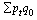. Index values can be derived from the corresponding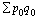. < Back

 Previous Page Next Page